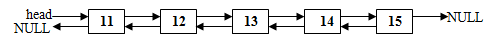Representation of a doubly linked list:

A doubly linked list contains backward as well as forward reference.  Each node contains two address along with the information field.  One address is the address of the next node and the other one is that of the previous node.  Thus doubly linked list is a bi-directional list, since we may traverse either from left to right or from right to left directly without any pointer manipulations as was the case with singly linked list.A doubly linked list may be represented using the following structure definition:

struct doublylist
{int info;
struct doublylist *next;
struct doublylist *prev;
};

Creation of a doubly linked list:  The program to create a doubly linked list is given below:

Program for operations on a Doubly Linked List

Operations covered in the following program:

• In-order traversal
• Reverse-order traversal
• Searching
• Insertion (inserting after, inserting before)
• Deletion (deleting after, deleting before)
• Disposing list

In-order traversal:  It refers to reading the elements of a doubly linked list from left to right.  The ‘C’ function for inorder traversal is as follows-

{
dlist *temp;
{printf(“List is empty.”);
}
else
{
while(temp!=NULL)
{printf(“%d->”,temp->info);
temp=temp->next;
}
printf(“bb “);
}
}

Reverse-order traversal:  Doubly linked list facilitates reverse order traversal, since reverse links are maintained via. keeping addresses of previous node as well as next node.  Thus a temp pointer may be positioned on the last element and then the traversal may begin from last to first using temp->prev navigation condition.  In the end, the first element may be printed.  The ‘C’ function is as follows:

{
dlist *temp;
{printf(“List is empty.”);
}
else
{
{
printf(“%d->”,temp->info);
temp=temp->prev;
}
printf(“%d->”,temp->info);
}
printf(“bb “);
}

Searching a node from a doubly linked list:  Searching a given value involves locating that value in the doubly list.  A temp pointer may be positioned at head node, and may be shifted until null is found or the given value is found.  The ‘C’ function to search a given node is as follows-

{
dlist *temp;
while(temp!=NULL)
{
if(temp->info==x)
return 1;
temp=temp->next;
}
return 0;
}

Insertion after a given node:  Inserting a new node after a given node requires four pointer adjustments.  First of all the node after which new node is to be inserted is to be located in the doubly list.  If it is found, then the following pointer assignments are required:

(i) The next address of new node must be set to the next of temp.

(ii) The prev address of new node must be set to temp.

(iv) Finally, the prev of next node of new node must be set to new node, if next node exists in the doubly list.

The ‘C’ function for the same is given below:

/*Insert a node after given node*/
{
dlist *temp,*ptr;
int ans;
if(ans==0)
{printf(“nNumber does not exist.”);
}
ptr=makenode(x);
while(temp->info!=val)
temp=temp->next;
ptr->next=temp->next;
ptr->prev=temp;
temp->next=ptr;
if(ptr->next!=NULL)
ptr->next->prev=ptr;
}

Insertion before a given node:  Insertion before a given node  is quite similar to that of insertion of a new node after a given node, provided we place temp pointer on the node before the node instead on that node (node before which new node is to be added up).  The ‘C’ functions that handles this operation is as under:

/*Insert a node before a given node*/
{
dlist *temp,*ptr;
int ans;
if(ans==0)
{printf(“nNumber does not exist.”);
}
ptr=makenode(x);
{
}
/*position temp on the node
before which to insert*/
while(temp->info!=val)
temp=temp->next;
ptr->next=temp;
ptr->prev=temp->prev;
temp->prev=ptr;
if(ptr->prev!=NULL)
ptr->prev->next=ptr;
}

Deletion after a given element/node:  To delete a node after a given node, locate that node in the doubly list.  Place temp pointer on that node.  Make following pointer assignments:

(i) Assign the next node of temp to any other pointer say ptr.

(ii) Assign the address in the next of temp as the address of next of ptr.

(iii) If a node after ptr exists, then assign the prev of next of ptr as temp.

(iv) Free pointer ptr.

The ‘C’ function is given below:

/*Delete node after a given node*/
{
dlist *temp,*ptr;
int ans;
if(ans==0)
{printf(“nNumber does not exist.”);
}
{printf(“nList is empty.”);
}
{printf(“nCannot delete. Only one node exists.”);
getch();
}
/*position temp at node after which to delete*/
while(temp->info!=x)
temp=temp->next;
ptr=temp->next;
temp->next=temp->next->next;
if(temp->next!=NULL)
temp->next->prev=ptr->prev;
free(ptr);
}

Deletion of a given element/node:  To delete the user defined node, locate that node such that the temp pointer is positioned on a node just before the node to be removed.  Then perform pointer adjustments as given in the previous case.  The ‘C’ function to delete a given node is as under:

{
dlist *temp,*ptr;
int ans;
if(ans==0)
{printf(“nNumber does not exist.”);
}

printf(“nList is empty.”);
else
while(temp->next->info!=x)
temp=temp->next;
ptr=temp->next;
temp->next=temp->next->next;
if(temp->next!=NULL)
temp->next->prev=ptr->prev;
free(ptr);
}
}

Deletion before a given element/node:  The function to delete a node before a given node is as follows:

/*delete node before a given node*/
{
dlist *temp,*ptr;
int ans;
if(ans==0)
{printf(“nNumber does not exist.”);
}
{printf(“nList is empty.”);
}
{printf(“nCannot delete. Only one node exists.”);
}
/*position temp at node before which to delete*/
while(temp->info!=x)
temp=temp->next;
ptr=temp->prev;
if(ptr->prev!=NULL)
{ptr->prev->next=temp;
temp->prev=ptr->prev;
}
else
{temp->prev=NULL;
}
free(ptr);
}

Disposing doubly linked list:  Disposing is the process of removing all the nodes of a doubly list and freeing the allocated memory, such that the list is finally deleted.  The ‘C’ function to dispose a doubly list is as follows:

/*disposing list*/